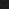JavaPerspective.com  >   Beginner Tutorials  >   5. Java in Practice  >   5.2. Reading user input

Last updated: 23 January 2013.

This tutorial will show you how to read input from the user through the command prompt (Windows users) or terminal (Linux and Mac users).

In the previous tutorial, you have learned how to pass data to an application at startup. However, in some situations, you may need to communicate more interactively with the user through the command prompt. For example, an application may prompt the user to enter his/her name this way:

The class UserInput shown below has a single method that simply reads the user's name from the standard input and prints it back to the standard output. If you are compiling and running Java programs with the command prompt (Windows) or terminal (Linux and Mac), by default, the standard input and output are both displayed in the command prompt or terminal.

public final class UserInput {

Scanner scanner =
new Scanner(System.in);
();

System.out.println
scanner.close
();

}
}

The field in of the class System is the standard input stream. Therefore, in order to read from the standard input stream, I passed System.in to the constructor of the class Scanner. I then used the method nextLine to read the user's name.

After reading the user's name, I used the operator + to concatenate the string value Hello and the user's name. Finally, I closed the scanner. The class App shown below contains a main method that calls the method readUserName:

public final class App {

public static void main(String[] args) {

}

}

The class Scanner was added to the Java API when Java 1.5 was released. Before Java 1.5, reading user input was not as simple as shown above. For example, the code below reads the user's name as it would be done before Java 1.5:

import java.io.IOException;

public final class UserInput {

();

System.out.println
();

}
}

The class App must be modified in order to handle the exception thrown by the method readUserName:

import java.io.IOException;

public final class App {

public static void main(String[] args) {

try{

}

catch(IOException e){

e.printStackTrace();

}
}

}

Code exercise 1

The idea is to write a program that reads an integer from the standard input using the class Scanner. If the user enters a string value that cannot be converted to an integer, the program prints an error message to the standard output and terminates. Otherwise, the program prints the integer to the standard output and terminates. Here is an example of valid input:

33
You have entered the number 33

And here is an example of invalid input:

3e
You did not enter a number

Write a method named readNumber in the class UserInput that reads an integer from the standard input:

import java.util.Scanner;

public final class UserInput {

private Scanner scanner = new Scanner(System.in);

// The code to read an integer goes here
// ...

}
}

Here is the class App that calls the method readNumber:

public final class App {

public static void main(String[] args) {

System.out.println
("You have entered the number " + number);

}

}

import java.util.Scanner;

public final class UserInput {

private Scanner scanner = new Scanner(System.in);

int result = 0;

try{

String number = scanner.nextLine
().trim();

result = Integer.parseInt
(number);

}

catch(NumberFormatException e){

System.out.println("You did not enter a number");
scanner.close
();
System.exit
(1);

}

return result;

}
}

Code exercise 2

Write a method named guessRandomNumber in the class UserInput that generates a random integer using the class Math. Then the method guessRandomNumber repeatedly asks the user to guess what the random number is. The program terminates when the user has found the random number. Here is an example:

45
45 is less than the random number
50
50 is greater than the random number
47
47 is greater than the random number
46
Congratulations ! You have found the random number !

You can use the method readNumber from the previous code problem (code exercise 1) to read an integer from the standard input. The random number is generated like this:

int randomNumber = ((int) (Math.random() * 100));

Here is the class App that calls the method guessRandomNumber:

public final class App {

public static void main(String[] args) {

new UserInput().guessRandomNumber();

}

}

import java.util.Scanner;

public final class UserInput {

private Scanner scanner = new Scanner(System.in);

public void guessRandomNumber(){

int randomNumber = ((int) (Math.random() * 100));

while(true){

if(enteredNumber < randomNumber){

System.out.println(enteredNumber + " is less than the random number");

}

else if(enteredNumber > randomNumber){

System.out.println(enteredNumber + " is greater than the random number");

}

else{

System.out.println("Congratulations ! You have found the random number !");

break;

}
}
}

int result = 0;

try{

String number = scanner.nextLine
().trim();

result = Integer.parseInt
(number);

}

catch(NumberFormatException e){

System.out.println("You did not enter a number");
scanner.close
();
System.exit
(1);

}

return result;

}
}

As you can see, the method readNumber is now declared private as it is not called from outside the class UserInput.

You are here :  JavaPerspective.com  >   Beginner Tutorials  >   5. Java in Practice  >   5.2. Reading user input
Next tutorial :  JavaPerspective.com  >   Beginner Tutorials  >   5. Java in Practice  >   5.3. Working with strings